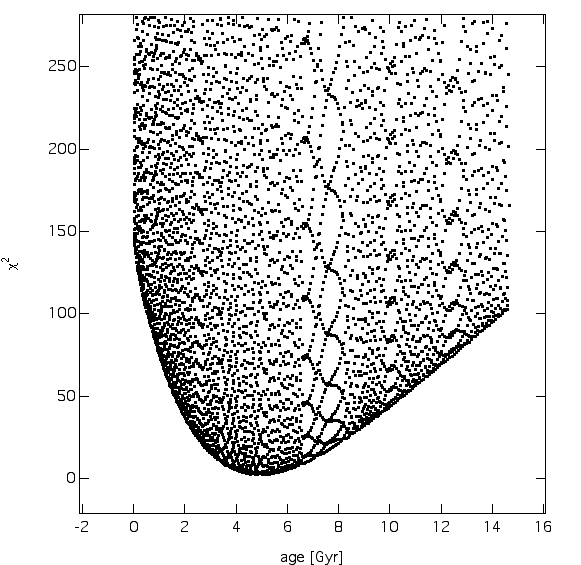## Problem

How to interpret a sparse (i.e., incomplete) set of low-l p-mode frequencies?

## Solution

Compare the observed oscillation spectrum to a vast and dense grid of stellar model oscillation spectra and pick out the closest match.

## Details

• Quantify the “closeness” of the match using chi-squared which also takes into account known uncertainties in stellar modeling and the observed frequencies.
• Grids of models ranging from 0.8 M_sun to 2.0 M_sun with mass resolution of 0.001 M_sun have been constructed using the Yale stellar evolution code.
• Models with Z=0.005 to Z=0.04 have so far been computed.
• Diffusion of helium and metals are included in some of the models, as is core overshoot.

## References

Guenther, D. B., 2004, “Quantitative Analysis of the Oscillation Spectrum of eta Boo,” ApJ, 612, 454

Guenther, D. B. and Brown, K. 2004, “Matching Stellar Models to Oscillation Data,” ApJ, 600, 419

## Figures

Figure 1. Quicktime Movie shows schematically the code searching for matches between model frequencies and the MOST observed frequencies.

Figure 2. Chi-squared measure for the Sun (BiSON data (l = 0, 1, and 2)). A well-defined minimum exists, which coincides with known age of Sun. I.e., the age of the sun is determined solely from the p-mode frequencies.Figure 3. Quicktime Movie showing chi-square results for alpha Cen (Bouchy & Carrier) ground based observations. Two distinct groups exist, one corresponding to models with convective cores and the other without.